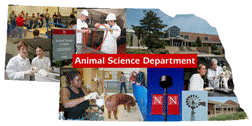Animal Science DepartmentFaculty Papers and Publications in Animal Science

Date of this Version

May 1965

Published in Journal of Dairy Science. Copyright © 1965 American Dairy Science Association. Used by permission.

Abstract

The regression of daughter on dam first-lactation milk deviations from herd-mate averages was found to be linear from an analysis of 47,400 Holstein pairs. The linear equation was y=-25.6 + .210 (x -27.6) and the quadratic equation was y=-25.6 + .208 (x -27.6) + .0000222 (x2-44131.4) with correlation coefficients, .18258 and .18268, respectively. These results suggest that selection response can be predicted adequately even with very large selection differentials.

COinS